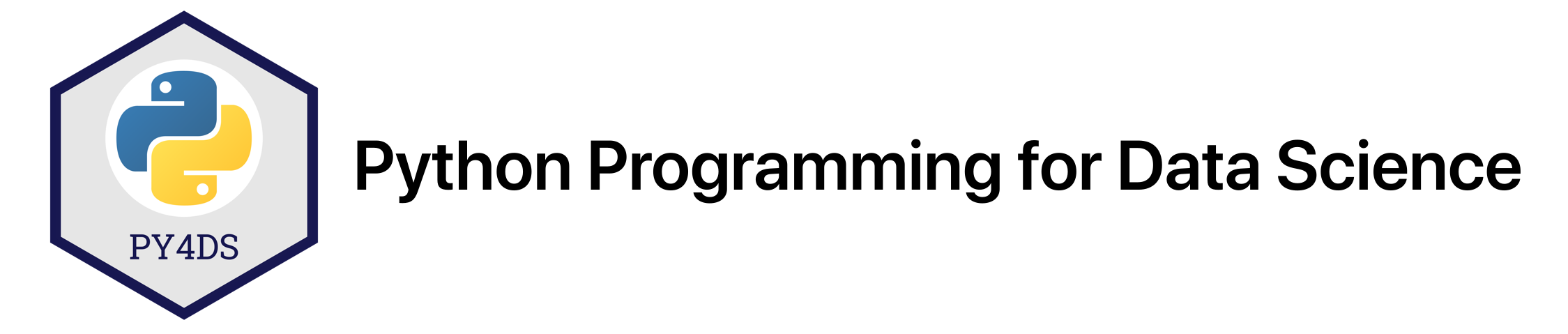# Loops & Functions¶

Tomas Beuzen, September 2020

These exercises complement Chapter 2.

## Exercises¶

### 1.¶

Create a function website() that grabs the website domain from a url string. For example, if your function is passed "www.google.com", it should return "google".

def website(url):
pass  # Remove this line and add your answer here.


### 2.¶

Create a function divisible(a, b) that accepts two integers (a and b) and returns True if a is divisble by b without a remainder. For example, divisible(10, 3) should return False, while divisible(6, 3) should return True.

def divisible(a, b):
pass  # Remove this line and add your answer here.


### 3.¶

Use list comprehension to square every number in the following list of numbers.

l = [1, 2, 3, 4, 5, 6, 7, 8, 9]



### 4.¶

For the following list of names, write a list comprehension that creates a list of only words that start with a capital letter (hint: str.isupper()).

names = ['Steve Irwin', 'koala', 'kangaroo', 'Australia', 'Sydney', 'desert']



### 5.¶

For the following list of keys and vals use dictionary comprehension to create a dictionary of the form {'key-0': 0, 'key-1': 1, etc} (hint: zip() can help you combine two lists into on object to be used for comprehension/looping).

keys = [f"key-{k}" for k in range(10)]
vals = range(10)



### 6.¶

This question is a little harder. Create a generator function called listgen(n) that yields numbers from 0 to n, in batches of lists of maximum 10 numbers at a time. For example, your function should behave as follows:

g = listgen(100)
next(g)
[0, 1, 2, 3, 4, 5, 6, 7, 8, 9]
next(g)
[10, 11, 12, 13, 14, 15, 16, 17, 18, 19]
next(g)
[20, 21, 22, 23, 24, 25, 26, 27, 28, 29]
etc.

g = listgen(5)
next(g)

def listgen(n):
pass  # Remove this line and add your answer here.


### 7.¶

Write a try/except to catch the error generated from the following code and print “I caught you!”. Make sure you catch the specific error being caused, this is typically better practice than just catching all errors!

5 / 0


---------------------------------------------------------------------------
ZeroDivisionError                         Traceback (most recent call last)
<ipython-input-7-9e2b7a365344> in <module>
----> 1 5 / 0
2

ZeroDivisionError: division by zero


### 8.¶

Create a function lucky_sum() that takes all the integers a user enters and returns their sum. However, if one of the values is 13 then it does not count towards the sum, nor do any values to its right.

For example, your function should behave as follows:

lucky_sum(1, 2, 3, 4)
10

lucky_sum(1, 13, 3, 4)
1

lucky_sum(13)
0


This example is inspired by the related codingbat challenge.

def lucky_sum(*args):
pass  # Remove this line and add your answer here.


## Solutions¶

### 1.¶

Create a function website() that grabs the website domain from a url string. For example, if your function is passed "www.google.com", it should return "google".

def website(url):
return url.split(".")

'google'


### 2.¶

Create a function divisible(a, b) that accepts two integers (a and b) and returns True if a is divisble by b without a remainder. For example, divisible(10, 3) should return False, while divisible(6, 3) should return True.

def divisible(a, b):
return True if a % b == 0 else False
print(divisible(10, 3))
print(divisible(6, 3))

False
True


### 3.¶

Use list comprehension to square every number in the following list of numbers.

l = [1, 2, 3, 4, 5, 6, 7, 8, 9]
[_ ** 2 for _ in l]

[1, 4, 9, 16, 25, 36, 49, 64, 81]


### 4.¶

For the following list of names, write a list comprehension that creates a list of only words that start with a capital letter (hint: str.isupper()).

names = ['Steve Irwin', 'koala', 'kangaroo', 'Australia', 'Sydney', 'desert']
[_ for _ in names if _.isupper()]

['Steve Irwin', 'Australia', 'Sydney']


### 5.¶

For the following list of keys and vals use dictionary comprehension to create a dictionary of the form {'key-0': 0, 'key-1': 1, etc} (hint: zip() can help you combine two lists into on object to be used for comprehension/looping).

keys = [f"key-{k}" for k in range(10)]
vals = range(10)
{k:v for k, v in zip(keys, vals)}

{'key-0': 0,
'key-1': 1,
'key-2': 2,
'key-3': 3,
'key-4': 4,
'key-5': 5,
'key-6': 6,
'key-7': 7,
'key-8': 8,
'key-9': 9}


### 6.¶

This question is a little harder. Create a generator function called listgen(n) that yields numbers from 0 to n, in batches of lists of maximum 10 numbers at a time. For example, your function should behave as follows:

g = listgen(100)
next(g)
[0, 1, 2, 3, 4, 5, 6, 7, 8, 9]
next(g)
[10, 11, 12, 13, 14, 15, 16, 17, 18, 19]
next(g)
[20, 21, 22, 23, 24, 25, 26, 27, 28, 29]
etc.

g = listgen(5)
next(g)

def listgen(n):
counter = 0
numbers = list(range(n))
while counter <= n // 10:
yield numbers[10 * counter:10*(counter+1)]
counter += 1


### 7.¶

Write a try/except to catch the error generated from the following code and print “I caught you!”. Make sure you catch the specific error being caused, this is typically better practice than just catching all errors!

try:
5 / 0
except ZeroDivisionError:
print("I caught you!")

I caught you!


### 8.¶

Create a function lucky_sum() that takes all the integers a user enters and returns their sum. However, if one of the values is 13 then it does not count towards the sum, nor do any values to its right.

For example, your function should behave as follows:

lucky_sum(1, 2, 3, 4)
10

lucky_sum(1, 13, 3, 4)
1

lucky_sum(13)
0


This example is inspired by the related codingbat challenge.

def lucky_sum(*args):
if 13 in args:
return sum(args[:args.index(13)])
return sum(args)